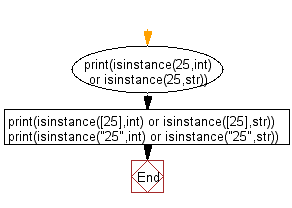﻿ Python: Check whether variable is integer or string - w3resource# Python: Check whether variable is integer or string

## Python Basic: Exercise-144 with Solution

Write a Python program to check whether a variable is integer or string.

Sample Solution :-

Python Code :

``````print(isinstance(25,int) or isinstance(25,str))
print(isinstance(,int) or isinstance(,str))
print(isinstance("25",int) or isinstance("25",str))
``````

Sample Output:

```True
False
True
```

Flowchart:## Visualize Python code execution:

The following tool visualize what the computer is doing step-by-step as it executes the said program:

Python Code Editor :

Have another way to solve this solution? Contribute your code (and comments) through Disqus.

What is the difficulty level of this exercise?

Test your Python skills with w3resource's quiz

﻿

## Python: Tips of the Day

Unpack variables from iterable:

```# One can unpack all iterables (tuples, list etc)
>>> a, b, c = 1, 2, 3
>>> a, b, c
(1, 2, 3)

>>> a, b, c = [1, 2, 3]
>>> a, b, c
(1, 2, 3)
```